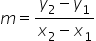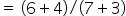Mathematics
Easy

Question

# Find the slope of the line that passes through (-3, -4) and (7, 6)

## 0Undefined-11Hint:

## The correct answer is: 1

### Points (-3, -4) and (7, 6).Step 1 : Slope of a line that passes through ( x1,y1), (x2,y2) isStep 2 : substituting the values= 6 -(-4) / 7 - (-3)=11 is required slope .

Given points ( x1,y1), (x2,y2)  the slope to pass through these points will be### Related Questions to study#### With Turito Foundation.#### Get an Expert Advice From Turito.